# Mathematics - Discrete Mathematics - Sets

in STEMGeekslast year (edited)[Image 1]

## Introduction

Hey it's a me again @drifter1!

Today we continue with Mathematics, and more specifically the branch of "Discrete Mathematics", in order to get into Sets. There will be multiple posts on them...

So, without further ado, let's get straight into it!

## Set Theory

Set Theory is all about studying collections of objects, known as sets, and can even be thought of as a complete branch of mathematics on its own. Such sets can be both discrete and continuous. In the context of discrete math, only the former will be discussed. The main concern will be how sets are arranged, combined and counted. These concepts, and sets in general actually, serve as a very important foundation for the mathematics used in discrete math.

## Sets

Sets are collections of distinct objects, which are of the same type. Each object in a set is commonly referred to as an element or member of that set.

### Set Representation

A set is denoted by a capital letter (A, B, ...) and the members by using lowercase letters (a, b, ...).

In tabular form, the elements of a set are enclosed in curly braces and separated by commas (,).

For example: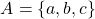### Common Notations

Commonly used notations include ∈, which is read as "is in" or "is an element of", and ∉, which is read as "is not in" or "is not an element of".

For example, a is in the set A, whilst d is not. This can be written mathematically as: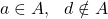A set with no elements, an empty set, is denoted by empty curly braces, { }, or the symbol ∅.

Other "special" sets which are useful in the context of discrete math are the sets of natural numbers and integers, respectively. They are denoted using a calligraphic N and Z, as shown below: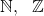### Set Builder Notation

Of course, if the elements are too many (maybe even infinite) the tabular form of notation mentioned previously is not sufficient. That's why sets can also be written as a list of properties that the elements should satisfy. This format is referred to as the set builder notation.

For example, all even natural numbers, can be written in respect to the natural number set, as follows: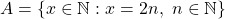The conditions can be written in "English" or completely in mathematical logic. There is no specifically defined way for writing them. It's common to have a colon (:), |, or even the special symbol ∍, which are all read as "such that", before the properties / conditions to be satisfied.

### Set Cardinality

For a finite set, the cardinality is the number of unique elements in that set. For a set A it's commonly denoted by |A|. This can also be extended for infinite sets, like the natural number set, which have basically infinite cardinality. So, the number of natural numbers and integers is basically the same, as both are countable infinite!

For example, set A in the previous example has a cardinality of 3, or: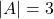The empty set ∅ has a cardinality of 0.

### Types of Sets

In addition to finite and infinite, sets can also be classified into subsets, proper subsets, improper subsets, and many more.

For two sets A and B, A is a subset of B, denoted by A ⊆ B, if all elements of A are also in B.

Two sets are equal, A = B, when they are subsets of each other, A ⊆ B and B ⊆ A. For an element x, this can also be written as: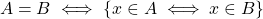If A ⊆ B, but A ≠ B, A is not equal to B, then A is a proper subset of B, which is denoted by A ⊂ B, which basically shows that not all elements of B are in A.

The empty set ∅ is a proper subset of every set.

In a similar fashion, if A ⊆ B and A = B, then A is an improper subset of B. So, every set is an improper subset of itself A ⊆ A.

And the list goes on...

• Sets with only one element are called singletons.

• Sets with equal cardinalities are equivalent sets.

• Sets with no common elements are disjoint sets.

## Exercise

Try to classify the following sets, as well as relate them with each other: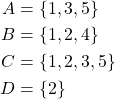The solution(s) will be in the comment section...

## RESOURCES:

### Images

Block diagrams and other visualizations were made using draw.io.

## Previous articles of the series

• Introduction → Discrete Mathematics, Why Discrete Math, Series Outline

## Final words | Next up

And this is actually it for today's post!

Next time we will continue on with Operations on Sets...

See ya!Keep on drifting!

Posted with STEMGeeks

Sort:

OMG, amazing post, recently I have been studying about Sets 😁😁

Solution to Exercise:

• All sets are finite sets
• Set D can be classified as a singleton set, because it has only one element
• Sets A and B have the same cardinality of 3 and are thus equivalent sets
• Set A is a proper subset of C, or A ⊂ C
• Set D is a proper subset of both B and C, and so D ⊂ B, D ⊂ C
• Sets A and D are disjoint, because they have no common elements